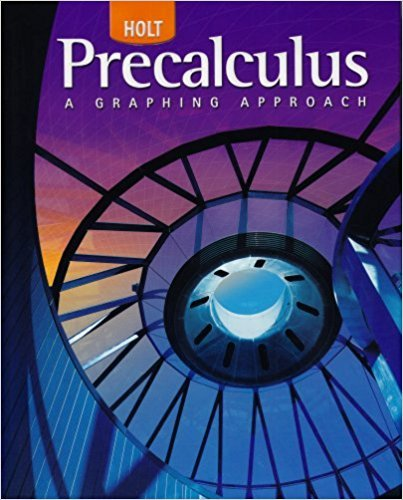×
×

# Solutions for Chapter 9.2 A: Excursion: Lines and Angles## Full solutions for Precalculus | 1st Edition

ISBN: 9780030416477Solutions for Chapter 9.2 A: Excursion: Lines and Angles

Solutions for Chapter 9.2 A
4 5 0 342 Reviews
21
5
##### ISBN: 9780030416477

Since 12 problems in chapter 9.2 A: Excursion: Lines and Angles have been answered, more than 24576 students have viewed full step-by-step solutions from this chapter. Precalculus was written by and is associated to the ISBN: 9780030416477. This expansive textbook survival guide covers the following chapters and their solutions. This textbook survival guide was created for the textbook: Precalculus, edition: 1. Chapter 9.2 A: Excursion: Lines and Angles includes 12 full step-by-step solutions.

Key Calculus Terms and definitions covered in this textbook
• Angular speed

Speed of rotation, typically measured in radians or revolutions per unit time

• Branches

The two separate curves that make up a hyperbola

• Cardioid

A limaçon whose polar equation is r = a ± a sin ?, or r = a ± a cos ?, where a > 0.

• Cosecant

The function y = csc x

• Dependent variable

Variable representing the range value of a function (usually y)

• Identity

An equation that is always true throughout its domain.

• Law of sines

sin A a = sin B b = sin C c

• Leibniz notation

The notation dy/dx for the derivative of ƒ.

• Linear factorization theorem

A polynomial ƒ(x) of degree n > 0 has the factorization ƒ(x) = a(x1 - z1) 1x - i z 22 Á 1x - z n where the z1 are the zeros of ƒ

• Product of matrices A and B

The matrix in which each entry is obtained by multiplying the entries of a row of A by the corresponding entries of a column of B and then adding

An angle in standard position whose terminal side lies on an axis.

• Range of a function

The set of all output values corresponding to elements in the domain.

• Solve algebraically

Use an algebraic method, including paper and pencil manipulation and obvious mental work, with no calculator or grapher use. When appropriate, the final exact solution may be approximated by a calculator

• Solve graphically

Use a graphical method, including use of a hand sketch or use of a grapher. When appropriate, the approximate solution should be confirmed algebraically

• Sum of an infinite series

See Convergence of a series

• Triangular number

A number that is a sum of the arithmetic series 1 + 2 + 3 + ... + n for some natural number n.

• Trichotomy property

For real numbers a and b, exactly one of the following is true: a < b, a = b , or a > b.

• Variable (in statistics)

A characteristic of individuals that is being identified or measured.

• Vertical translation

A shift of a graph up or down.

• Xmin

The x-value of the left side of the viewing window,.

×